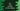# C++ program to find the GCD of two numbers## C++ program to find the GCD of two numbers:

GCD or greatest common divisor is the largest number that can divide both numbers. It is also called HCF of two numbers. We can find the GCD in many ways. In this post, we will learn how to find the GCD of two numbers in different ways in C++.

### Method 1: By using a for loop:

Using a for loop, we can find the GCD of two numbers.

``````#include <iostream>
using namespace std;

int main()
{
int first, second, i, gcd;

cout << "Enter the first number : " << endl;
cin >> first;

cout << "Enter the second number : " << endl;
cin >> second;

for (i = 1; i <= first && i <= second; i++)
{
if (first % i == 0 && second % i == 0)
{
gcd = i;
}
}

cout << "GCD : " << gcd << endl;
}``````

Here,

• We are taking the first and the second number and storing it in first and second.
• The for loop runs from i = 1 and checks if a number can divide both first and second properly or not. If yes, it assigns that value to gcd.
• Finally, it printed the value of gcd.

It will give output as like below:

``````Enter the first number :
18
Enter the second number :
1251
GCD : 9``````

### Method 2: Reverse traversal:

This method is more efficient that the above method. Here, we will use the for loop to traverse in reverse and find out the gcd. The for loop will start from the number which is smaller and run till 1. If it find any number that can divide both first and second, it will exit from the loop. Because, that will be the largest number that can divide both, no need to check for other smaller values.

Let’s take a look at the program:

``````#include <iostream>
using namespace std;

int main()
{
int first, second, i, gcd;

cout << "Enter the first number : " << endl;
cin >> first;

cout << "Enter the second number : " << endl;
cin >> second;

for (i = first > second ? second : first; i >= 0; i--)
{
if (first % i == 0 && second % i == 0)
{
gcd = i;
break;
}
}

cout << "GCD : " << gcd << endl;
}``````
• This is almost similar to the above program.
• The only difference is that i starts from the smaller value between first and second and ends at 1.
• If a GCD is found, it exit from the loop and prints that value.

It will give similar output.

### Method 3: Using while loop:

We can also find GCD using a while loop. The idea is to keep subtracting the smaller number from the larger number and assigning that value to that number until both numbers are equal. That is the GCD or HCF of the numbers.

``````#include <iostream>
using namespace std;

int main()
{
int first, second;

cout << "Enter the first number : " << endl;
cin >> first;

cout << "Enter the second number : " << endl;
cin >> second;

while(first != second){
if(first > second){
first = first - second;
}else{
second = second - first;
}
}

cout << "GCD : " << first << endl;
}``````

This will print a similar output to the user.